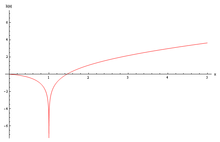# Logarithmic integral function

﻿
Logarithmic integral function

In mathematics, the logarithmic integral function or integral logarithm li(x) is a special function. It occurs in problems of physics and has number theoretic significance, occurring in the prime number theorem as an estimate of the number of prime numbers less than a given value.Logarithmic integral function plot

## Integral representation

The logarithmic integral has an integral representation defined for all positive real numbers$x\ne 1$ by the definite integral:${\rm li} (x) = \int_0^x \frac{dt}{\ln t}. \;$

Here, ln denotes the natural logarithm. The function 1 / ln(t) has a singularity at t = 1, and the integral for x > 1 has to be interpreted as a Cauchy principal value:${\rm li} (x) = \lim_{\varepsilon \to 0+} \left( \int_0^{1-\varepsilon} \frac{dt}{\ln t} + \int_{1+\varepsilon}^x \frac{dt}{\ln t} \right). \;$

## Offset logarithmic integral

The offset logarithmic integral or Eulerian logarithmic integral is defined as${\rm Li}(x) = {\rm li}(x) - {\rm li}(2) \,$

or${\rm Li} (x) = \int_2^x \frac{dt}{\ln t} \,$

As such, the integral representation has the advantage of avoiding the singularity in the domain of integration.

This function is a very good approximation to the number of prime numbers less than x.

## Series representation

The function li(x) is related to the exponential integral Ei(x) via the equation$\hbox{li}(x)=\hbox{Ei}(\ln x) , \,\!$

which is valid for x > 1. This identity provides a series representation of li(x) as${\rm li} (e^u) = \hbox{Ei}(u) = \gamma + \ln u + \sum_{n=1}^\infty {u^{n}\over n \cdot n!} \quad \text{ for } u \ne 0 \; ,$

where γ ≈ 0.57721 56649 01532 ... is the Euler–Mascheroni gamma constant. A more rapidly convergent series due to Ramanujan  is${\rm li} (x) = \gamma + \ln \ln x + \sqrt{x} \sum_{n=1}^\infty \frac{ (-1)^{n-1} (\ln x)^n} {n! \, 2^{n-1}} \sum_{k=0}^{\lfloor (n-1)/2 \rfloor} \frac{1}{2k+1} .$

## Special values

The function li(x) has a single positive zero; it occurs at x ≈ 1.45136 92348 ...; this number is known as the Ramanujan–Soldner constant.

li(2) ≈ 1.045163 780117 492784 844588 889194 613136 522615 578151…

This is$-(\Gamma\left(0,-\ln 2\right) + i\,\pi)$ where$\Gamma\left(a,x\right)$ is the incomplete gamma function. It must be understood as the Cauchy principal value of the function.

## Asymptotic expansion

The asymptotic behavior for x → ∞ is${\rm li} (x) = O \left( {x\over \ln x} \right) \; .$

where O is the big O notation. The full asymptotic expansion is${\rm li} (x) \sim \frac{x}{\ln x} \sum_{k=0}^\infty \frac{k!}{(\ln x)^k}$

or$\frac{{\rm li} (x)}{x/\ln x} \sim 1 + \frac{1}{\ln x} + \frac{2}{(\ln x)^2} + \frac{6}{(\ln x)^3} + \cdots.$

Note that, as an asymptotic expansion, this series is not convergent: it is a reasonable approximation only if the series is truncated at a finite number of terms, and only large values of x are employed. This expansion follows directly from the asymptotic expansion for the exponential integral.

## Infinite logarithmic integral

[clarification needed]:$\int_{-\infty}^\infty \frac{M(t)}{1+t^2}dt$

and discussed in Paul Koosis, The Logarithmic Integral, volumes I and II, Cambridge University Press, second edition, 1998.

## Number theoretic significance

The logarithmic integral is important in number theory, appearing in estimates of the number of prime numbers less than a given value. For example, the prime number theorem states that:$\pi(x)\sim\operatorname{li}(x)$

where π(x) denotes the number of primes smaller than or equal to x.

Wikimedia Foundation. 2010.

### Look at other dictionaries:

• Integral logarithm — The term integral logarithm may stand for: * Discrete logarithm in algebra, * Logarithmic integral function in calculus …   Wikipedia

• Logarithmic differentiation — Logarithmic derivative is a separate article. Topics in Calculus Fundamental theorem Limits of functions Continuity Mean value theorem Differential calculus  Derivative Change of variables Implicit differentiation …   Wikipedia

• Logarithmic mean — In mathematics, the logarithmic mean is a function of two numbers which is equal to their difference divided by the logarithm of their quotient. In symbols::egin{matrix}M {mbox{lm(x,y) =lim {(xi,eta) o(x,y)} frac{eta xi}{ln eta ln xi}… …   Wikipedia

• Logarithmic derivative — In mathematics, specifically in calculus and complex analysis, the logarithmic derivative of a function f is defined by the formula where f ′ is the derivative of f. When f is a function f(x) of a real variable x, and takes real, strictly… …   Wikipedia

• Logarithmic scale — A logarithmic scale is a scale of measurement that uses the logarithm of a physical quantity instead of the quantity itself. Presentation of data on a logarithmic scale can be helpful when the data covers a large range of values ndash; the… …   Wikipedia

• Exponential integral — is a mathematical function of a single argument which has no established notation.DefinitionsFor real values of x , the exponential integral Ei( x ) can be defined as : mbox{Ei}(x)=int { infty}^xfrac{e^t}t,dt., The definition above can be used… …   Wikipedia

• Prime-counting function — In mathematics, the prime counting function is the function counting the number of prime numbers less than or equal to some real number x cite book |first=Eric |last=Bach |coauthors=Shallit, Jeffrey |year=1996 |title=Algorithmic Number Theory… …   Wikipedia

• Landau's function — g ( n ) is defined for every natural number n to be the largest order of an element of the symmetric group S n . Equivalently, g ( n ) is the largest least common multiple of any partition of n .For instance, 5 = 2 + 3 and lcm(2,3) = 6. No other… …   Wikipedia

• Trigonometric integral — Si(x) (blue) and Ci(x) (green) plotted on the same plot. In mathematics, the trigonometric integrals are a family of integrals which involve trigonometric functions. A number of the basic trigonometric integrals are discussed at the list of… …   Wikipedia

• Gamma function — For the gamma function of ordinals, see Veblen function. The gamma function along part of the real axis In mathematics, the gamma function (represented by the capital Greek letter Γ) is an extension of the factorial function, with its… …   Wikipedia